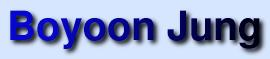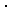Boyoon Jung's Library Manual

bj::mrkd_node Struct Reference

Data structure for a node of a multiresolution KD-tree. More...

#include <kd_tree.h>

Collaboration diagram for bj::mrkd_node:[legend]
List of all members.

Public Attributes

int split_dim
A splitting dimension.
double split_val
A splitting value.
int num_points
The number of points owned by this sub-tree.
gsl_vector * hr_min
The bounding hyper-rectangle of the points below this node.
gsl_vector * hr_max
The bounding hyper-rectangle of the points below this node.
gsl_vector * centroid
The centroid of the points owned by this leaf node.
gsl_matrix * cov
The covariance of the points owned by this leaf node.
mrkd_nodeleft
A left mrkd-tree.
mrkd_noderight
A right mrkd-tree.

Detailed Description

Data structure for a node of a multiresolution KD-tree.

Member Data Documentation

 gsl_vector* bj::mrkd_node::centroid
 The centroid of the points owned by this leaf node.

 gsl_matrix* bj::mrkd_node::cov
 The covariance of the points owned by this leaf node.

 gsl_vector* bj::mrkd_node::hr_max
 The bounding hyper-rectangle of the points below this node.

 gsl_vector* bj::mrkd_node::hr_min
 The bounding hyper-rectangle of the points below this node.

 A left mrkd-tree.

 The number of points owned by this sub-tree.

 A right mrkd-tree.

 A splitting dimension.

 double bj::mrkd_node::split_val
 A splitting value.

The documentation for this struct was generated from the following file:
ResearchProjectsPublications

ToolsBjLib ManualPlayer/StagePyPlayerMicroStrain IMUGPS/DGPSParticle Filter

PersonalWelcomeCurriculum VitaeResumeContactUSCUSC CS DeptCRESRobotics LabRESL